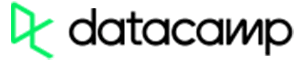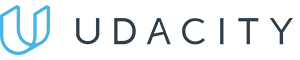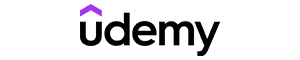Code has been added to clipboard!

# Using the Python round Function

Published Feb 13, 2020
Updated Feb 13, 2020

TL;DR – The built-in Python round function allows you to round numbers.

## How to round in Python

The Python `round` function rounds a floating point number to a specified amount of decimals:

Example
``````number = round(37.8452950483520, 4)
print(number)

number2 = round(37.8452950483520, 2)
print(number2)

number3 = round(37.8452950483520)
print(number3)``````

You can also use Python `round` for negative numbers:

Example
``````number = round(-14.573883749392, 4)
print(number)

number2 = round(-14.573883749392, 2)
print(number2)

number3 = round(-14.573883749392)
print(number3)``````Pros
• Easy to use with a learn-by-doing approach
• Offers quality content
• Gamified in-browser coding experience
• The price matches the quality
• Suitable for learners ranging from beginner to advanced
Main Features
• Free certificates of completion
• Focused on data science skills
• Flexible learning timetablePros
• Simplistic design (no unnecessary information)
• High-quality courses (even the free ones)
• Variety of features
Main Features
• Nanodegree programs
• Suitable for enterprises
• Paid Certificates of completionPros
• Easy to navigate
• No technical issues
• Seems to care about its users
Main Features
• Huge variety of courses
• 30-day refund policy
• Free certificates of completion

## The syntax for round in Python

The Python `round` function takes up to two arguments:

• A floating point number to round
• A number of decimals to round the number to

The second argument is optional: if you skip it, the number will be rounded to the nearest integer (no decimals). If using both, you have to separate the arguments with a comma:

``round(float, decimals)``

## Python round: useful tips

• Make sure you define both arguments for rounding in Python in numbers – otherwise, a TypeError will fire.
• In some cases, you might get inconsistent results for example, `2.75` is rounded to `2.8`, but `2.65` is rounded to `2.6`. It's not a bug: the system simply cannot represent some decimal fractions exactly as floats.

Days
Hours
Minutes
Seconds